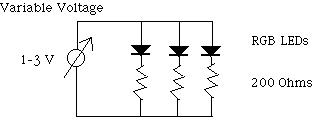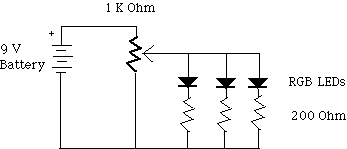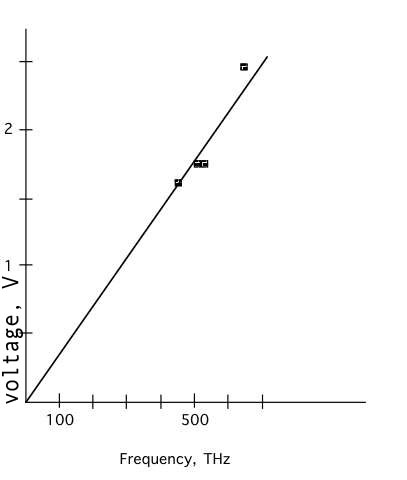Light Energy and Color with LED’s

Blue light photons have higher energy than red

Introduction

Light emitting diodes, LEDs, convert the energy change of one electron into one light photon. Slowly increase the energy of the electrons by increasing the voltage across the LEDs and you will see that the red LED comes on at the lowest voltage then the green and finally the blue at the highest voltage.

Material

• A red, green, and blue LED (All available from Radio Shack)
• 3 x 200 ohm resistors
• A voltmeter (You will measure voltages between 1 and 3 volts)
• Either an adjustable DC voltage supply (1-3 volts or more)
• or a 9 volt battery and holder
• and a 1K ohm potentiometer
• The circuit can be assembled on the Rathjen breadboard.

Assembly

Connect the LED’s in series with the 200 ohm resistors.
Connect the LED’s and resistors in parallel then connect them to the variable voltage supply.Or, if you wish to use the 9 volt battery, then build a voltage divider. Connect the 1 k
W potentiometer to the 9 volt battery, then connect the diodes with their series resistors in from the output of the potentiometer to ground.To Do and Notice

Slowly increase the voltage across the LED’s.
Notice that first the red LED comes on, then the green and finally the blue.
Then repeat the process of raising the voltage.
When you first see light in the red LED use the voltmeter to measure the voltage across the LED.
When the green LED first comes on, measure the voltage across its leads.
When the blue LED comes on, measure the voltage across its leads.

What’s Going On?

A light emitting diode changes the electrical energy of one electron into the light energy of one photon. Thus lower energy photons of red light are produced when the electrons drop through smaller voltage differences while higher energy blue photons require higher voltage differences. It is interesting to see the lights come on in order, first red then green then blue

Incandescent bulbs convert the energy change of one electron as it crosses a resistance into more than, or fewer than one photon.

More detail

The negative electrons have high energy at the negative terminal of the battery. In the LED they enter the N-type semiconductor and drop all of their energy at the junction with a P-type semiconductor as they fall into holes. This energy is emitted as electromagnetic radiation, visible light in LEDs. The electrons then return to the positive terminal of the battery. In an incandescent bulb the electrons drop their energy gradually as they travel through the filament. Their electrical energy is converted to thermal energy. This thermal energy is then converted into electromagnetic radiation.

Math Root

The energy, E, in joules, of a photon is proportional to its frequency, f, in hertz.

E = hf

Where h is the constant of proportionality known as Planck's constant.
h =
6.6 x 10^-34 J s

Red light has a frequency near 500 THz, (500 Terahertz, 500 x 10^12 Hz)
Green near 600 THz
Blue near 750 THz

So the energy of a red photon is E = 6.6 x 10^-34 x 500 x 10^12 = 3.3 x 10^-19 J

and the blue E = 5 x 10^-19 J.

You can plot the Voltage for each LED versus the frequencyRecall the equation relating the energy of a photon, E, to its frequency, f, and the equation relating the energy of an electron of charge q, to its voltage, V.:

E = hf and E = qV

an LED makes these equal so the line represents

qV = hf

the slope of the voltage versus frequency line is then

V = h/q f

The slope is Planck's constant divided by the charge on an electron q which is 1.6 x 10^-19 C

The slope should be 6.6 x 10^-34/1.6 x 10^-19 or about 4 * 10^-15 Js/C or 4 V/GHz

(A GHz is 1000 THz)

The slope of our line is about 3 Volts per GHz

Not a bad measurement for under \$10.

Etc

You can look at the light produced by the LEDs through a diffraction grating. You will see that the deflection of the image of the lamp is proportional to the wavelength of the light, the longer wavelength red is deflected more than the shorter wavelength blue. A commercial spectroscope can be calibrated in light frequency or energy so that the frequency of the light from each LED can be read off the spectroscope.

The spectroscope will give the wavelength of the light not its frequency. To convert wavelength, L, to frequency, f, you will need to calculate c = fL, where c is the speed of light 3 x 10^8 m/s.